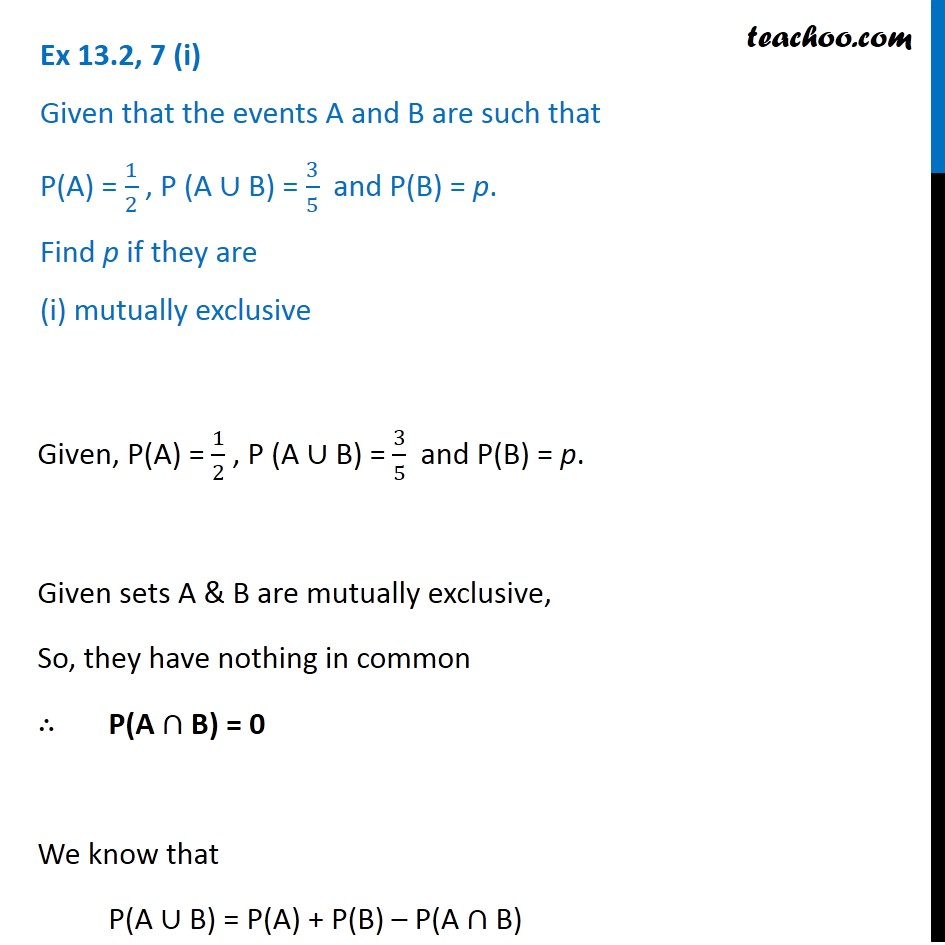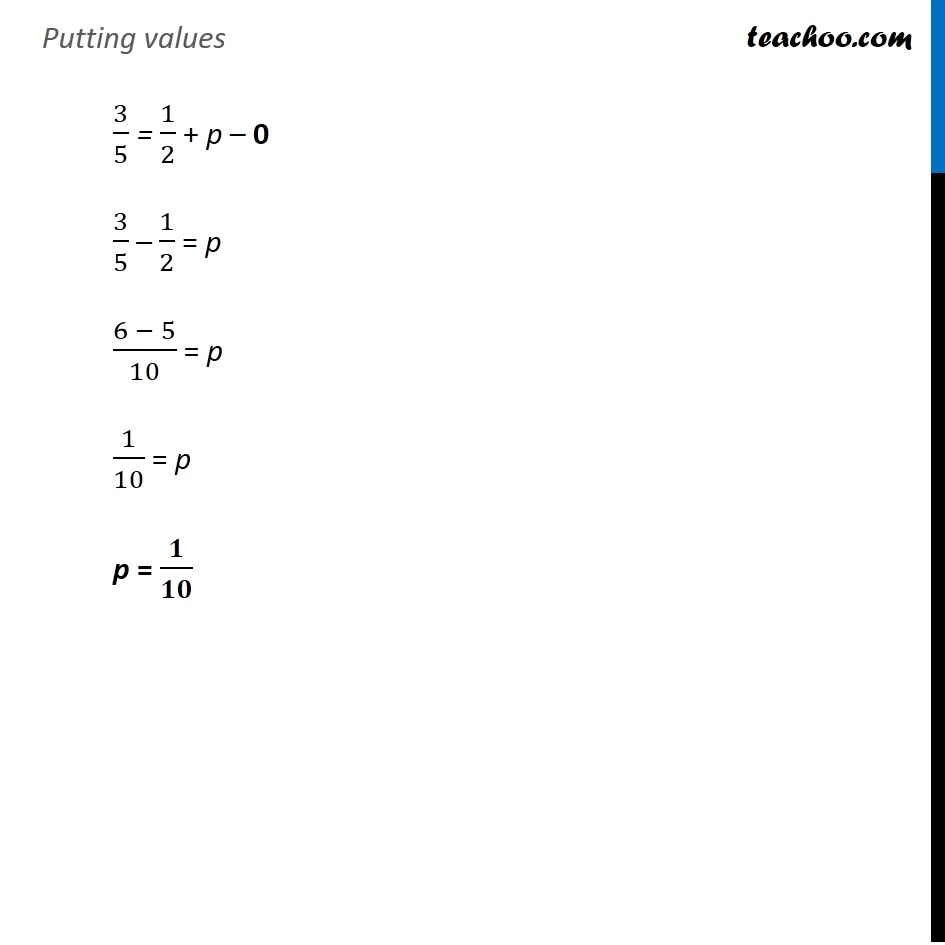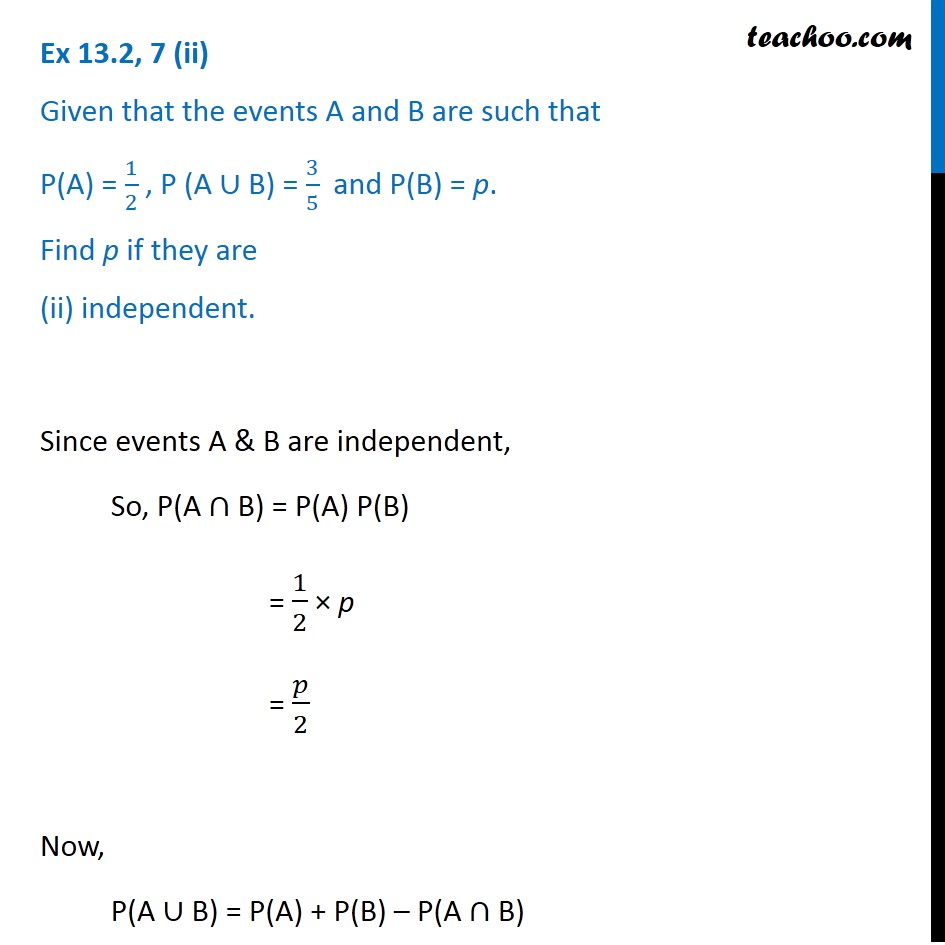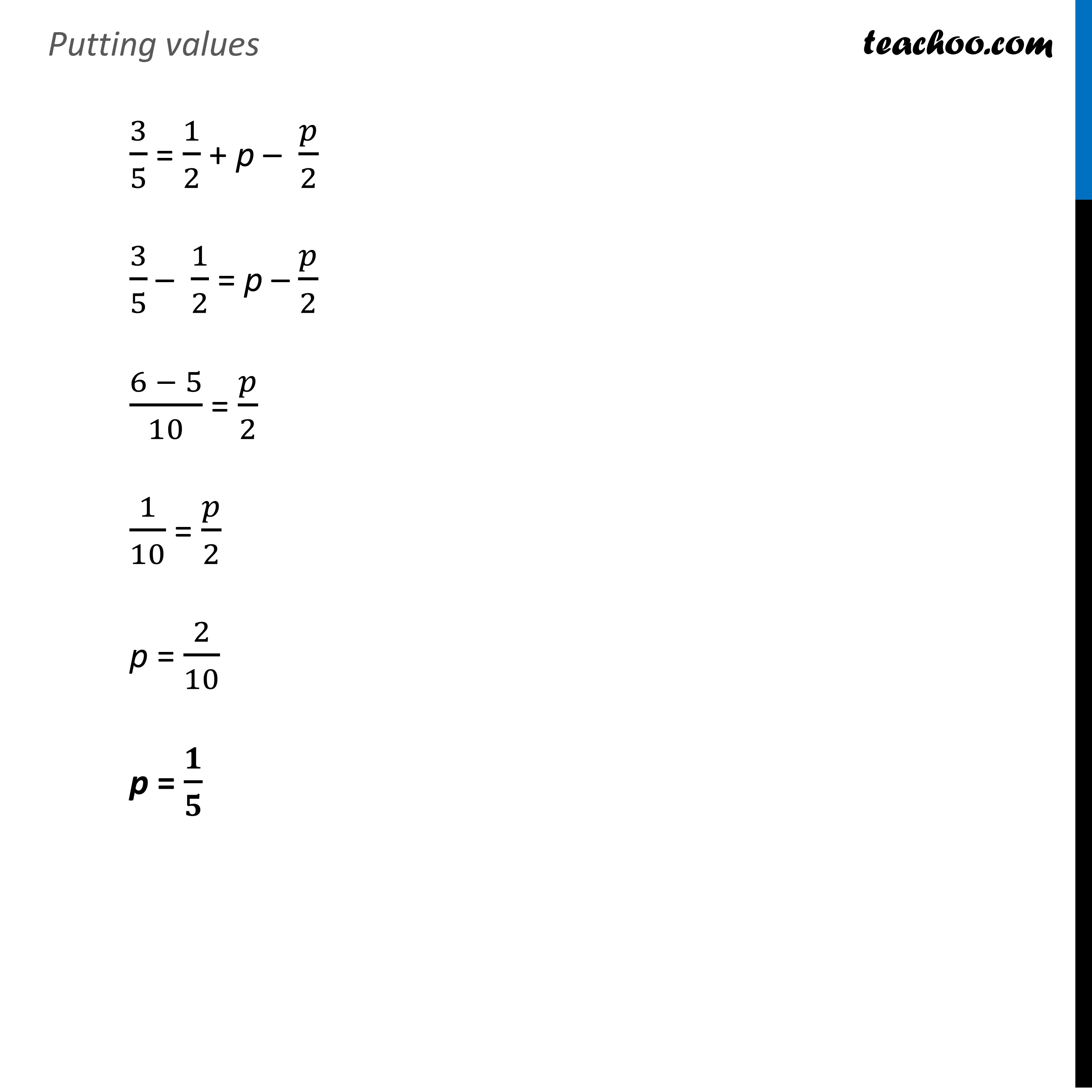Independent events

Chapter 13 Class 12 Probability
Concept wiseLearn in your speed, with individual attention - Teachoo Maths 1-on-1 Class

### Transcript

Ex 13.2, 7 (i) Given that the events A and B are such that P(A) = 1/2 , P (A ∪ B) = 3/5 and P(B) = p. Find p if they are (i) mutually exclusiveGiven, P(A) = 1/2 , P (A ∪ B) = 3/5 and P(B) = p. Given sets A & B are mutually exclusive, So, they have nothing in common ∴ P(A ∩ B) = 0 We know that P(A ∪ B) = P(A) + P(B) – P(A ∩ B) Putting values 3/5 = 1/2 + p – 0 3/5 – 1/2 = p (6 − 5)/10 = p 1/10 = p p = 𝟏/𝟏𝟎 Ex 13.2, 7 (ii) Given that the events A and B are such that P(A) = 1/2 , P (A ∪ B) = 3/5 and P(B) = p. Find p if they are (ii) independent.Since events A & B are independent, So, P(A ∩ B) = P(A) P(B) = 1/2 × p = 𝑝/2 Now, P(A ∪ B) = P(A) + P(B) – P(A ∩ B) Putting values 3/5 = 1/2 + p – 𝑝/2 3/2 – 1/2 = p – 𝑝/2 (6 − 5)/10 = 𝑝/2 1/10 = 𝑝/2 p = 2/10 p = 𝟏/𝟓